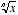index: click on a letter A B C D E F G H I J K L M N O P Q R S T U V W X Y Z A to Z index index: subject areas numbers & symbols sets, logic, proofs geometry algebra trigonometry advanced algebra & pre-calculus calculus advanced topics probability & statistics real world applications multimedia entrieswww.mathwords.com about mathwords website feedback

 nth Root The number that must be multiplied times itself n times to equal a given value. The nth root of x is writtenor. For example,since 25 = 32. Notes: When n = 2 an nth root is called a square root. Also, if n is even and x is negative, thenis nonreal.     See also